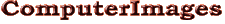Support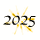# Keeping Time with Excel.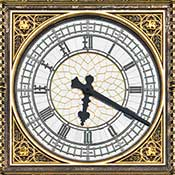To Excel 6:20 p.m. is 0.763888888888889.1

Ever need to compute that a workday that begins at 8:30 AM and ends at 4:00 PM is 8½ hours? Or that a 5-hour delivery that ships at 8:15 AM should arrive at 1:15 PM? Or that a lab process took 1 minute 12 seconds based on recorded starting and ending times? Excel can do all of that for you.

How It Works. Time calculations are a combination of simple arithmetic and formatting. The arithmetic simply adds, subtracts, multiplies and divides ordinary numbers. The formatting displays the entries and computed results as hours, minutes and seconds rather than decimal numbers.

Excel calculates time as fractions of a day. A 24-hour day is represented by the number 1. An hour is 1/24th of that or 0.041667 and a minute is 1/60th of an hour or 0.000694. Obviously those numbers would be confusing, so Excel can format them as something familiar, such as hh:mm:ss.

Excel begins counting hours of the day at midnight with zero. Since 6:00 AM is a quarter of the way into the day its value is 0.25. The preceding table gives examples.

Time calculations are easy so long as they set up correctly. You need to focus on three things:

• Making sure Excel understands which entries are times,
• Setting up the formulas correctly, and
• Formatting results so they are meaningful.

How Excel Reads Time Entries. Excel doesn’t understand every entry that looks like a time as such. Those it doesn’t are treated as text and time calculations based on them don’t work.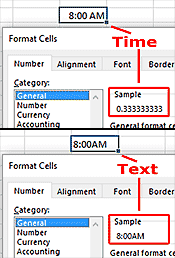“General” Format Samples for Time and Text Entries.

When Excel reads an entry as time, it records it as a fraction of a day and automatically switches to a time format. The table above shows entries that Excel recognizes as time. Even the slightest deviation matters. For example, Excel reads 8:00 AM as time, but 8:00AM and 8:00 A.M. as text.

There is an easy way to see how Excel interprets a cell entry. Right click on the cell, choose “Format Cell” from the menu, click the “Number” tab at the top of the dialogue box and select the “General” format category. If the sample is a decimal number, Excel reads the entry as time. If it’s the same as you see in the cell, Excel reads it as text.

If you are working with imported times that Excel reads as text, you probably can use text and time functions to convert them into Excel-readable times. Look on-line for techniques suggested for your specific data.2

Time Calcu­la­tions. It is easy to calculate the duration between two times, just subtract the start time from the finish time. The table shows examples for different entries in cells A1 and B1.

Obviously the last result is not what we want — the duration from 9:00 PM to 1:00 AM is four hours. This error occurs whenever an event goes from PM to AM since Excel’s number for 9:00 PM is greater than its number for 1:00 AM and therefore produces a negative result when subtracted. There is a simple solution: The formula =MOD(B1-A1,1) gives the correct result.3

Adding or subtracting hours and minutes to a start or finish time is a little more complicated since Excel would evaluate the simple entry +5 or -5 in a formula as meaning five days. To add 5 hours, you can do either of the following:

• Format the cell containing the hours and minutes to be added or subtracted as time and enter the amount as hh:mm. Then just add or subtract that cell to the cell containing the start or finish time (see examples in table).
• Use Excel’s TIME function to convert numbers to time values. For example, TIME(5,0,0) means 5 hours, no minutes and no seconds, thus if cell A1 contains 8:00 AM, the formula =A1+TIME(5,0,0) would produce the result 1:00 PM.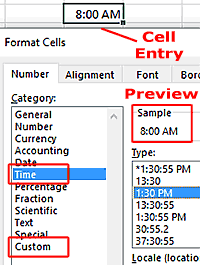Time Format Options in Format Cells Dialogue Box.

Formatting Times. None of us would want to calculate time in Excel if we had to read the results as fractions of a day. So Excel provides number formats that display input entries and computed results as hours and minutes, or times of day.

To Excel the value 0.75 means ¾ day and is used both for the duration 18 hours and the time 6:00 PM. We need to choose the format that displays what we want. Excel has several standard time formats and gives us the ability to create our own custom formats.

The standard settings are in the Format Cells dialogue box. To use them, select the cells to format, right-click and choose Format Cells from the menu. Go to the “Number” tab and click “Time” in the category list. Choices are displayed on the right. The sample previews how each will display your data.

You can also enter custom format codes if none of the built-in options gives exactly what you want. To do this, choose "Custom" at the bottom of the category list and enter the codes for the format you want in the box on the right. The symbols “h”, “m” and “s” represent hours, minutes and seconds. Entering “hh” versus “h” indicates that you want leading zeros included. The table gives samples for the time value 0.2502.

The last four examples show use of square brackets to prevent Excel from displaying 60 minutes as one hour as it normally would. Thus where the exact value is 6 hours and 17 seconds, [m] displays 360 minutes and [s] displays 21617 seconds. The difference between [m].00 and [m]:ss is that [m].00 displays decimal fractions of a minute while [m]:ss displays seconds.

Date and Time. Excel stores dates as serial numbers, with January 1, 1900 being day 1. See Dates with Excel. If you specify a time, Excel adds the decimal value for that time to the date’s serial number. For example, May 15, 2017 is day 42870. Thus noon on that day is 42870.5.

-----

1. Image of Big Ben from photo by David Iliff posted on Wikipedia.
2. Articles include Neil Rubenking, Easy Date and Time Entry in Excel, PC Magazine (June 1, 2008) and How to convert time string to time in Excel?, ExtendOffice web site.
3. The formula in essence computes the remainder of dividing the time difference by 1. It works because where the time difference is negative the MOD function subtracts it from 1 giving the correct result. Don’t use the MOD function if the entries contain both date and time, e.g. 5/15/2017 10:00 PM. It’s not needed and will produce wrong results.